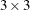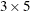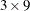# The X11 Procedure

### MACURVES Statement

• MACURVES month=option …;

The MACURVES statement specifies the length of the moving-average curves for estimating the seasonal factors for any month. This statement can be used only with monthly time series data.

The month=option specifications consist of the month name (or the first three letters of the month name), an equal sign, and one of the following option values:

’3’

specifies a three-term moving average for the month

’3X3’

specifies a three-by-three moving average

’3X5’

specifies a three-by-five moving average

’3X9’

specifies a three-by-nine moving average

STABLE

specifies a stable seasonal factor (average of all values for the month)

For example, the statement

   macurves jan='3' feb='3x3' march='3x5' april='3x9';


uses a three-term moving average to estimate seasonal factors for January, a(a three-term moving average of a three-term moving average) for February, a(a three-term moving average of a five-term moving average) for March, and a(a three-term moving average of a nine-term moving average) for April.

The numeric values used for the weights of the various moving averages and a discussion of the derivation of these weights are given in Shiskin, Young, and Musgrave (1967). A general discussion of moving average weights is given in Dagum (1985).

If the specification for a month is omitted, the X11 procedure uses a three-by-three moving average for the first estimate of each iteration and a three-by-five average for the second estimate.